Question

If one wants to test whether there is a difference in mean scores before and after an exam, which will be an appropriate test to use?

a) one sample z test

b) one sample t test

c) paired t test
d) two sample independent t test

If X is a random variable following a Uniform(a,b) distribution, which option represents an approxi- mate shape of its density function?

a) straight vertical line between a and b

b) straight horizontal line between a and b

c) symmetric U shaped curve between a and b

3. LetX∼N(20,1). WhatisP(X>20)?

a) 0.25

b) 0.5

c) 0.75

d) 0.99

If the data is in the form of a frequency table, it is best described using a:

a) histogram

b) pie-chart

c) stem and leaf plot

d) boxplot

1. Answer: Option C paired t test

To compare difference between population means before and after an trial or experiment, we use paired t-test

2. Answer: Option C Symmetric U shaped curve between a and b

Since it is continuous uniform distribution with values lies between a and b, hence frequency curve will be U shaped with values symmetrically distributed between range a and b

3. Answer: Option B 0.5

X ~ N(20,1)

P(x>20) = P[(x - 20) / 1 > (20 - 20) / 1]=P(z > 0) = 1-P(z < 0) = 1 - 0.5 = 0.5

4. Answer: Option A Histogram

Histogram helps in identifying distribution of data represented in frequency table i.e whether data is unimodal (one mode value) or bimodal (exists two mode values) or symmetrical or skewed as well as if it is skewed then whether it is positively or negative skewed.

#### Earn Coins

Coins can be redeemed for fabulous gifts.

Similar Homework Help Questions
• ### 1. If one wants to test whether there is a difference in mean scores before and...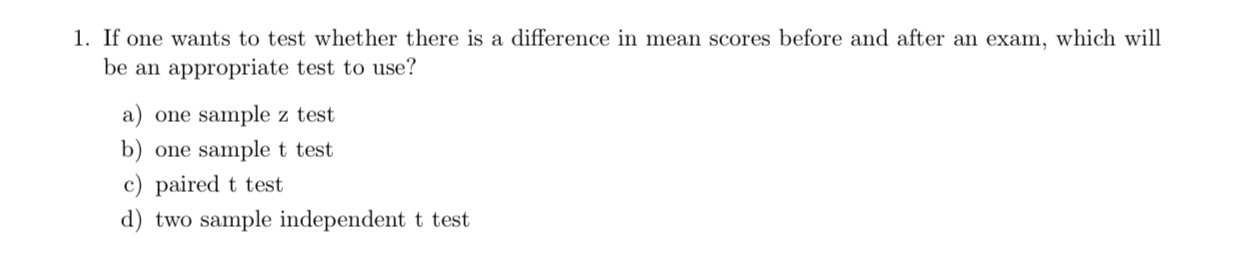1. If one wants to test whether there is a difference in mean scores before and after an exam, which will be an appropriate test to use? a) one sample z test b) one sample t test c) paired t test d) two sample independent t test

• ### Multiple Choice Questions Choose the one alternative that best completes the statement or answers the question...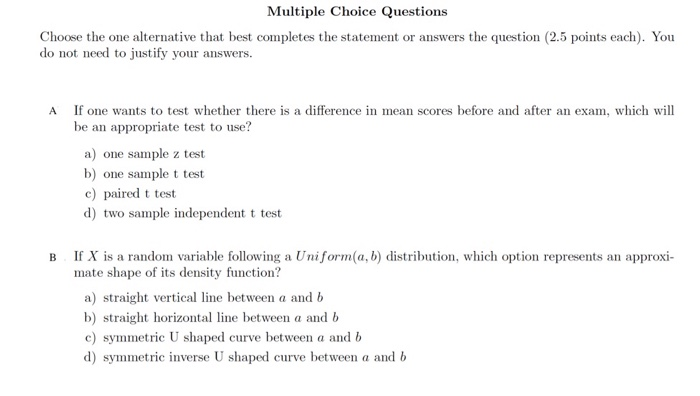Multiple Choice Questions Choose the one alternative that best completes the statement or answers the question (2.5 points each). You do not need to justify your answers. A If one wants to test whether there is a difference in mean scores before and after an exam, which will be an appropriate test to use? a) one sample z test b) one sample t test c) paired t test d) two sample independent t test B If X is a random...

• ### A. Let X ~ N(20,1). What is P(X > 20) ? a) 0.25 b) 0.5 c)...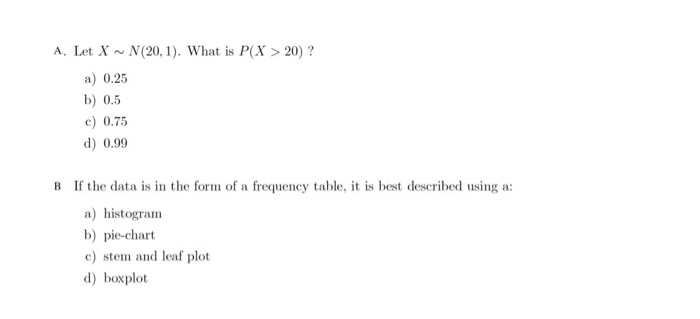A. Let X ~ N(20,1). What is P(X > 20) ? a) 0.25 b) 0.5 c) 0.75 d) 0.99 B If the data is in the form of a frequency table, it is best described using a: a) histogram b) pie-chart c) stem and leaf plot d) boxplot

• ### 2. If X is a random variable following a Uniform(a,b) distribution, which option represents an approxi-...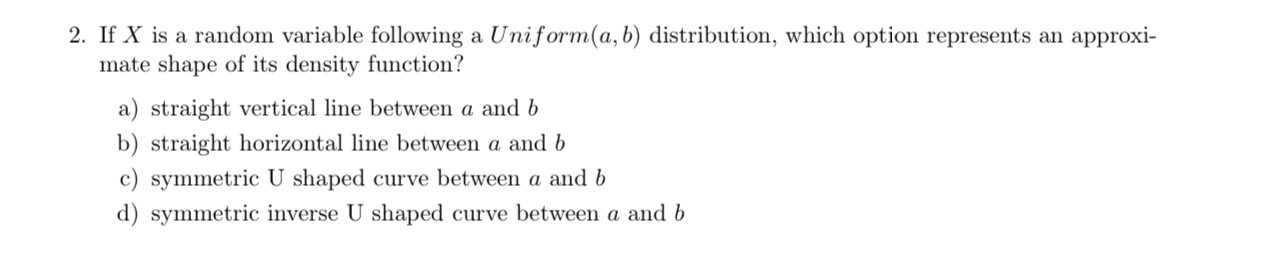2. If X is a random variable following a Uniform(a,b) distribution, which option represents an approxi- mate shape of its density function? a) straight vertical line between a and b b) straight horizontal line between a and b c) symmetric U shaped curve between a and b d) symmetric inverse U shaped curve between a and b

• ### If X is a random variable following a Uniform(a,b) distribution, which option represents an approxi- mate...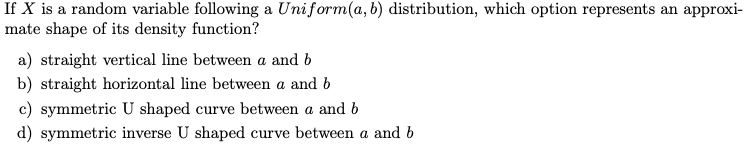If X is a random variable following a Uniform(a,b) distribution, which option represents an approxi- mate shape of its density function? a) straight vertical line between a and b b) straight horizontal line between a and b c) symmetric U shaped curve between a and b d) symmetric inverse U shaped curve between a and b

• ### The boxplot of the exam scores for the students in an introductory statistics course is given...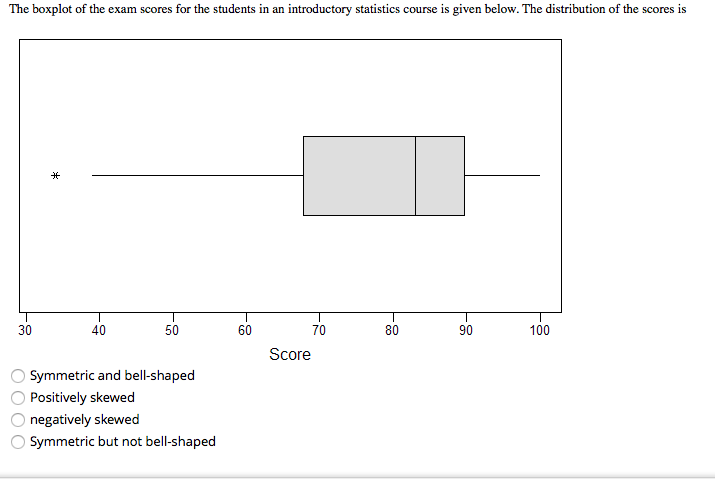The boxplot of the exam scores for the students in an introductory statistics course is given below. The distribution of the scores is 30 40 50 60 70 Score 80 90 100 Symmetric and bell-shaped Positively skewed O negatively skewed Symmetric but not bell-shaped A Ph.D. graduate has applied for a job with two universities: A and B. The graduate feels that she has a 60% chance of receiving an offer from university A and a 50% chance of receiving...

• ### 1- Choose the functions that should be used to test whether your dataset meets the assumptions...

1- Choose the functions that should be used to test whether your dataset meets the assumptions for a two-sample t-test. Select one or more: a. mean() b. normalTest() c. qt() d. var.test() e. var() 2- Which of the following is the name of a mandatory argument to the function read.csv in R? Select one: a. file b. sep c. header d. quote 3- Match the type of comparison to its appropriate null hypothesis. The sample mean is not different from...

• ### please answer all question 16-20 Question 16 2 pts The population mean for a standardized test...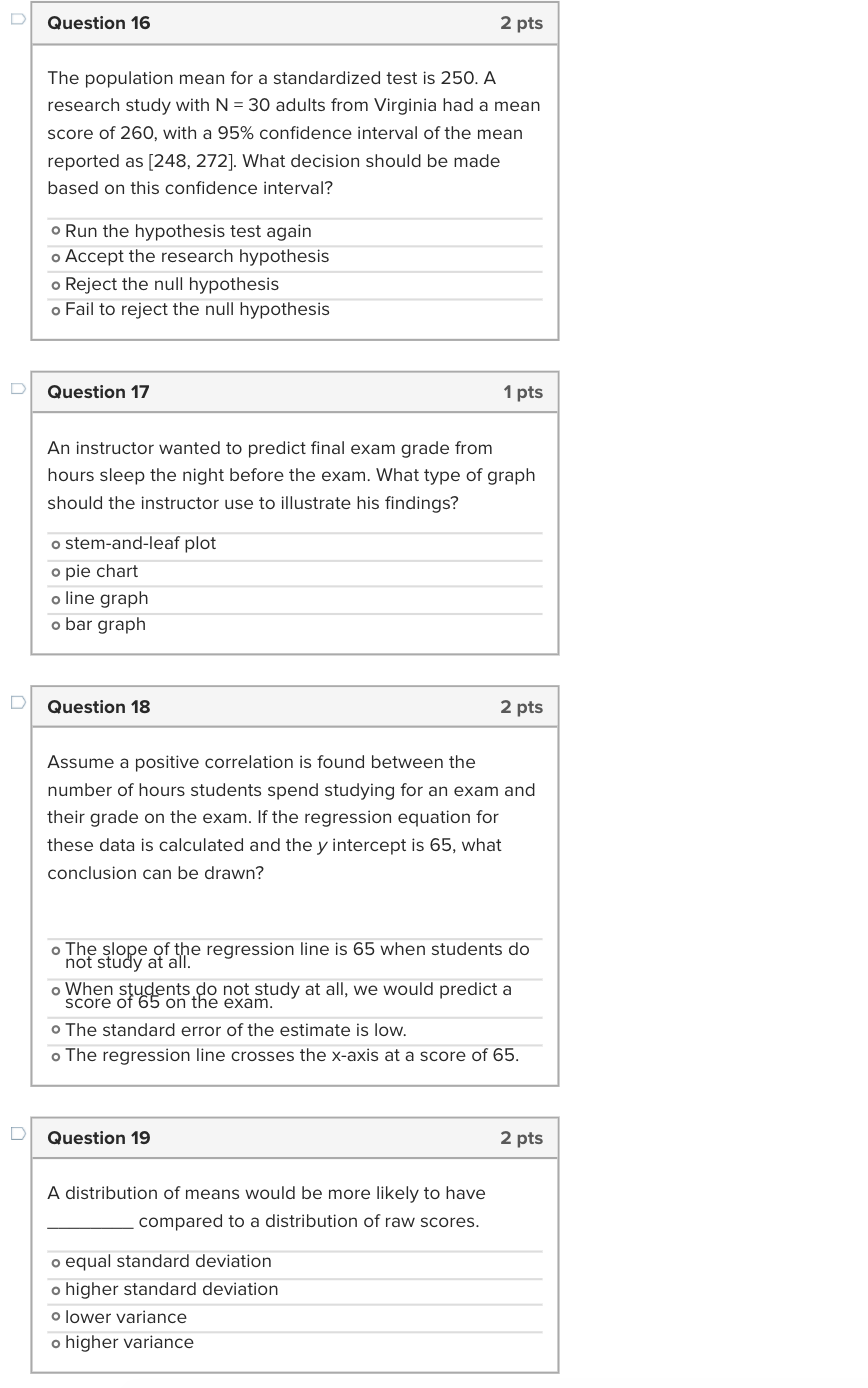please answer all question 16-20 Question 16 2 pts The population mean for a standardized test is 250. A research study with N = 30 adults from Virginia had a mean score of 260, with a 95% confidence interval of the mean reported as [248, 272]. What decision should be made based on this confidence interval? o Run the hypothesis test again o Accept the research hypothesis o Reject the null hypothesis o Fail to reject the null hypothesis Question...

• ### 21) A researcher wants to know whether there is a difference in the physical activity level of children during the morni...

21) A researcher wants to know whether there is a difference in the physical activity level of children during the morning and afternoon. He observes a total of 40 children (20 boys and 20 girls) from randomly selected schools during morning and afternoon recess and gives each child a score (out of 50) based on how much of the time they spend sitting, standing, walking or running. Each child receives a score in the morning and another in the afternoon....

• ### To test Upper H 0H0​: muμequals=100 versus Upper H 1H1​: muμnot equals≠​100, a simple random sample...

To test Upper H 0H0​: muμequals=100 versus Upper H 1H1​: muμnot equals≠​100, a simple random sample size of nequals=1717 is obtained from a population that is known to be normally distributed. Answer parts​ (a)-(d). LOADING... Click here to view the​ t-Distribution Area in Right Tail. ​(a) If x overbarxequals=104.4104.4 and sequals=8.78.7​, compute the test statistic. tequals=2.0852.085 ​(Round to three decimal places as​ needed.)​(b) If the researcher decides to test this hypothesis at the alphaαequals=0.01 level of​ significance, determine the critical...